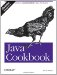# Chapter 5. Numbers

 Introduction Section 5.1.  Checking Whether a String Is a Valid Number Section 5.2.  Storing a Larger Number in a Smaller Number Section 5.3.  Converting Numbers to Objects and Vice Versa Section 5.4.  Taking a Fraction of an Integer Without Using Floating Point Section 5.5.  Ensuring the Accuracy of Floating-Point Numbers Section 5.6.  Comparing Floating-Point Numbers Section 5.7.  Rounding Floating-Point Numbers Section 5.8.  Formatting Numbers Section 5.9.  Converting Between Binary, Octal, Decimal, and Hexadecimal Section 5.10.  Operating on a Series of Integers Section 5.11.  Working with Roman Numerals Section 5.12.  Formatting with Correct Plurals Section 5.13.  Generating Random Numbers Section 5.14.  Generating Better Random Numbers Section 5.15.  Calculating Trigonometric Functions Section 5.16.  Taking Logarithms Section 5.17.  Multiplying Matrices Section 5.18.  Using Complex Numbers Section 5.19.  Handling Very Large Numbers Section 5.20.  Program: TempConverter Section 5.21.  Program: Number PalindromesJava Cookbook, Second Edition
ISBN: 0596007019
EAN: 2147483647
Year: 2003
Pages: 409
Authors: Ian F Darwin

Similar book on Amazon